Before you go through this article, make sure that you have gone through the previous article on Ripple Carry Adder.

• Each full adder has to wait for its carry-in from its previous stage full adder.
• Thus, nth full adder has to wait until all (n-1) full adders have completed their operations.
• This causes a delay and makes ripple carry adder extremely slow.
• The situation becomes worst when the value of n becomes very large.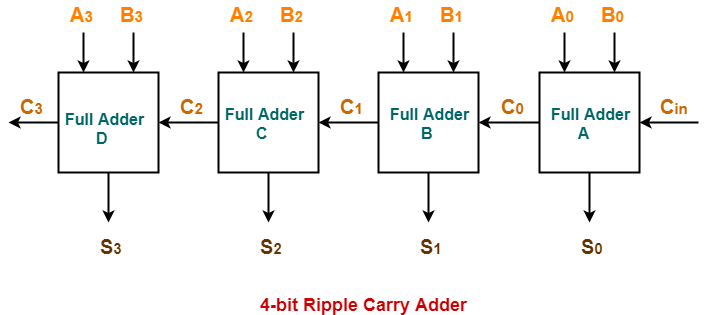• It generates the carry-in of each full adder simultaneously without causing any delay.
• The time complexity of carry look ahead adder = Θ (logn).

## Logic Diagram-

The logic diagram for carry look ahead adder is as shown below-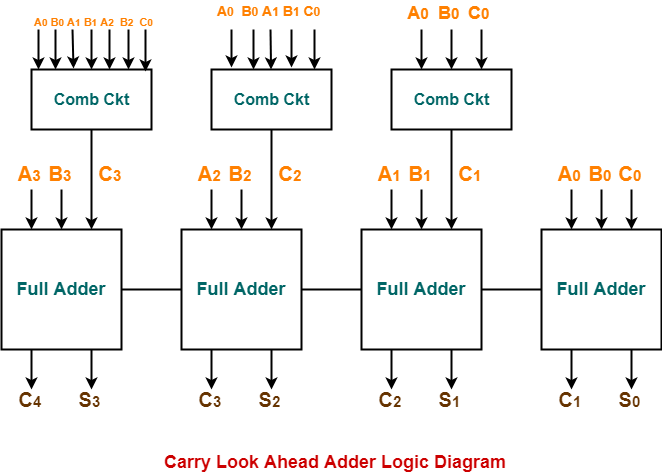The working of carry look ahead adder is based on the principle- The carry-in of any stage full adder is independent of the carry bits generated during intermediate stages.

The carry-in of any stage full adder depends only on the following two parameters-

• Bits being added in the previous stages
• Carry-in provided in the beginning

Now,

• The above two parameters are always known from the beginning.
• So, the carry-in of any stage full adder can be evaluated at any instant of time.
• Thus, any full adder need not wait until its carry-in is generated by its previous stage full adder.

Consider two 4-bit binary numbers A3A2A1A0 and B3B2B1B0 are to be added.

Mathematically, the two numbers will be added as-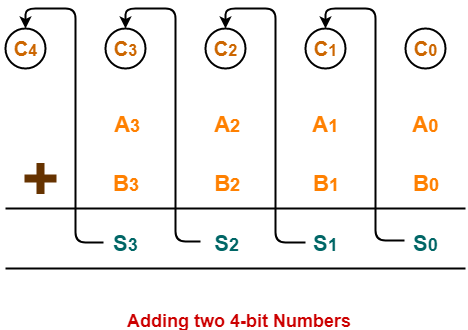From here, we have-

C1 = C0 (A0 ⊕ B0) + A0B0

C2 = C1 (A1 ⊕ B1) + A1B1

C3 = C2 (A2 ⊕ B2) + A2B2

C4 = C3 (A3 ⊕ B3) + A3B3

For simplicity, Let-

• Gi = AiBi where G is called carry generator
• Pi = Ai ⊕ Bi where P is called carry propagator

Then, re-writing the above equations, we have-

C1 = C0P0 + G………….. (1)

C2 = C1P1 + G1 ………….. (2)

C3 = C2P2 + G2 ………….. (3)

C4 = C3P3 + G3 ………….. (4)

Now,

• Clearly, C1, C2 and C3 are intermediate carry bits.
• So, let’s remove C1, C2 and C3 from RHS of every equation.
• Substituting (1) in (2), we get C2 in terms of C0.
• Then, substituting (2) in (3), we get C3 in terms of C0 and so on.

Finally, we have the following equations-

• C1 = C0P0 + G
• C2 = C0P0P1 + G0P1 + G1
• C3 = C0P0P1P2 + G0P1P2 + G1P2 + G2
• C4 =C0P0P1P2P3 + G0P1P2P3 + G1P2P3 + G2P3 + G3

These equations are important to remember.

These equations show that the carry-in of any stage full adder depends only on-

• Bits being added in the previous stages
• Carry bit which was provided in the beginning

### Trick To Memorize Above Equations-

As an example, let us consider the equation for generating carry bit C2.

There are three possible reasons for generation of C2 as depicted in the following picture-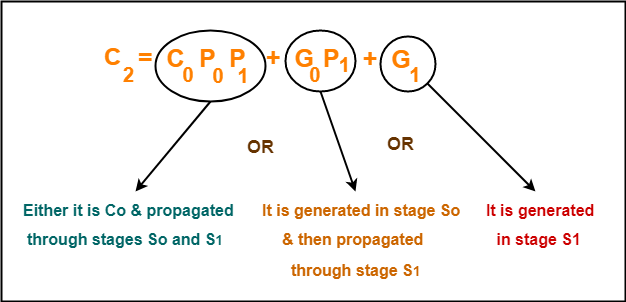In the similar manner, we can write other equations as well very easily.

## Implementation Of Carry Generator Circuits-

The above carry generator circuits are usually implemented as-

• Two level combinational circuits.
• Using AND and OR gates where gates are assumed to have any number of inputs.

### Implementation Of C1–

• The carry generator circuit for C1 is implemented as shown below.
• It requires 1 AND gate and 1 OR gate.

C1 = C0P0 + G0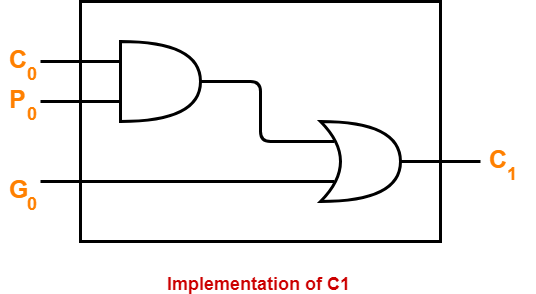### Implementation Of C2–

• The carry generator circuit for C2 is implemented as shown below.
• It requires 2 AND gates and 1 OR gate.

C2 = C0P0P1 + G0P1 + G1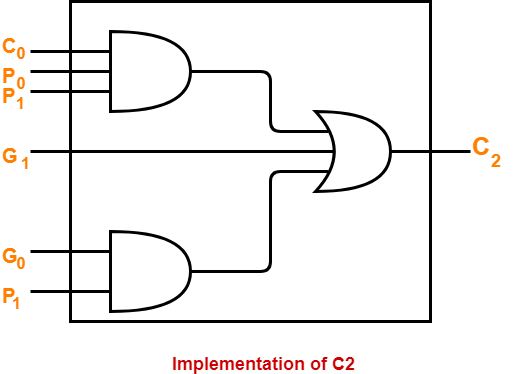### Implementation Of C3 & C4–

Similarly, we implement C3 and C4.

• Implementation of C3 uses 3 AND gates and 1 OR gate.
• Implementation of C4 uses 4 AND gates and 1 OR gate.

Total number of gates required to implement carry generators (provided carry propagators Pi and carry generators Gi) are-

• Total number of AND gates required for addition of 4-bit numbers = 1 + 2 + 3 + 4 = 10.
• Total number of OR gates required for addition of 4-bit numbers = 1 + 1 + 1 + 1 = 4.

### General Formula-

The following formula is used to calculate number of gates required for evaluating all carry bits-

 For a n-bit carry look ahead adder to evaluate all the carry bits, it requires- Number of AND gates = n(n+1) / 2 Number of OR gates = n

• It generates the carry-in for each full adder simultaneously.
• It reduces the propagation delay.

• It involves complex hardware.
• It is costlier since it involves complex hardware.
• It gets more complicated as the number of bits increases.

Watch this Video Lecture

Next Article- K Maps | Karnaugh Maps

Get more notes and other study material of Digital Design.

Watch video lectures by visiting our YouTube channel LearnVidFun.

Before you go through this article, make sure that you have gone through the previous article on Ripple Carry Adder.

We have discussed-

• Ripple Carry Adder is a combinational logic circuit.
• It is used for the purpose of adding two n-bit binary numbers.
• It is also called as n-bit parallel adder.## Delay in Ripple Carry Adder-

Consider a N-bit Ripple Carry Adder as shown-

##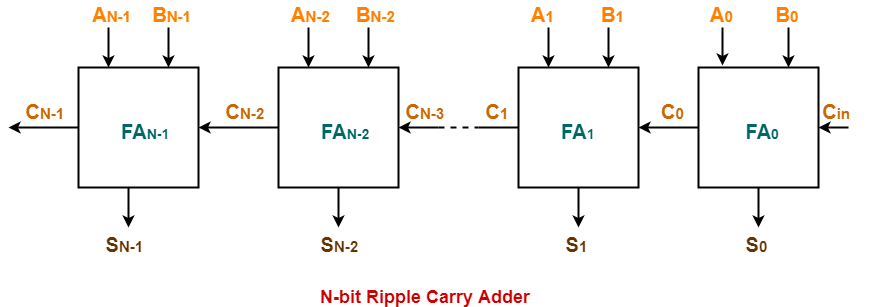The following kinds of problems may be asked based on delay calculation in Ripple Carry Adder.

## Type-01 Problem:

• You will be given the carry propagation delay and sum propagation delay of each full adder.
• You will be asked to calculate the worst case delay of the ripple carry adder.

## Solution-

### Know These Terms?

It is important to know the following terms-

• Carry propagation delay of a full adder is the time taken by it to produce the output carry bit.
• Sum propagation delay of a full adder is the time taken by it to produce the output sum bit.
• Worst case delay of a ripple carry adder is the time after which the output sum bit and carry bit becomes available from the last full adder.

• When carry in becomes available to the full adder, it starts its operation.
• It produces the corresponding output sum bit and carry bit.

If you are asked to calculate the time after which the output sum bit or carry bit becomes available from any particular full adder, then it is calculated as-

### Time After Which Carry Bit Cx Becomes Available-

Required time

= Total number of full adders till full adder producing Cx X Carry propagation delay of full adder

### Time After Which Sum Bit Sx Becomes Available-

Required time

= Time taken for its carry in to become available + Sum propagation delay of full adder

= { Total number of full adders before full adder producing Sx X Carry propagation delay of full adder } + Sum propagation delay of full adder

We will calculate worst case delay for the last full adder.

## Type-02 Problem:

• You will be given the propagation delay of some basic logic gates.
• You will be told how the full adder has been implemented.
• Then, you will be asked to calculate the worst case delay of Ripple Carry Adder.

Suppose each full adder in the given ripple carry adder has been implemented as-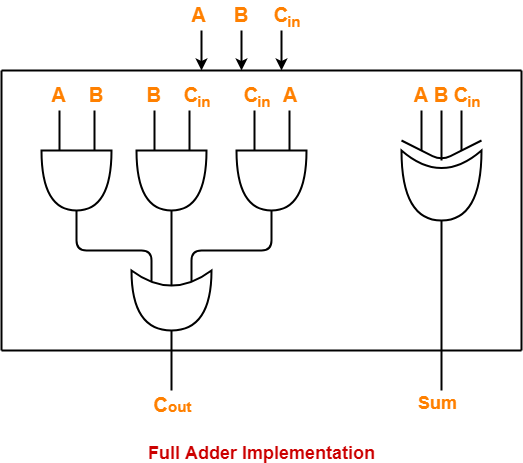## Solution-

• The computation has to be done in the same manner as in Type-01 problem.
• It’s just that in Type-02 problem, one step is increased.
• We have to first calculate the carry propagation delay and sum propagation delay in terms of logic gates.
• Then, our problem will reduce to Type-01 problem.

Let-

• Propagation delay of AND gate = Tpd (AND)
• Propagation delay of OR gate = Tpd (OR)
• Propagation delay of XOR gate = Tpd (XOR)

### Calculating Carry Propagation Delay-

• We calculate the carry propagation delay of full adder using its carry generator logic circuit.
• It has 2 levels in the given implementation.
• At first level, three AND gates operate.
• All the three AND gates operate in parallel.
• So, we consider the propagation delay due to only one AND gate.
• At second level, OR gate operates.

Now,

Carry propagation delay of full adder

= Time taken by it to generate the output carry bit

= Propagation delay of AND gate + Propagation delay of OR gate

= Tpd (AND) + Tpd (OR)

### Calculating Sum Propagation Delay-

• We calculate the sum propagation delay of full adder using its sum generator logic circuit.
• It has only 1 level at which XOR gate operates in the given implementation.

Now,

Sum propagation delay of full adder

=  Time taken by it to generate the output sum bit

= Propagation delay of XOR gate

= Tpd (XOR)

Now,

• We have got the carry propagation delay and sum propagation delay of full adders.
• Our problem reduces to Type-01 problem.
• We use the same formulas as we have learnt in Type-01 problem to make the required calculations.

### NOTE-

Consider in the question,

• It was said that while implementing the sum generator logic circuit of full adders, only 2-input XOR gates are used.
• Then, in that case we would require two such XOR gates which would work at 2 levels.
• So, in that case, sum propagation delay would be twice the propagation delay of XOR gate.

## Problem-01:

A 16-bit ripple carry adder is realized using 16 identical full adders. The carry propagation delay of each full adder is 12 ns and the sum propagation delay of each full adder is 15 ns. The worst case delay of this 16 bit adder will be ______?

A) 195 ns

B) 220 ns

C) 250 ns

D) 300 ns

## Solution-

We consider the last full adder for worst case delay.

Time after which output carry bit becomes available from the last full adder

= Total number of full adders X Carry propagation delay of full adder

= 16 x 12 ns

= 192 ns

Time after which output sum bit becomes available from the last full adder

= Time taken for its carry in to become available + Sum propagation delay of full adder

= { Total number of full adders before last full adder X Carry propagation delay of full adder } + Sum propagation delay of full adder

= { 15 x 12 ns } + 15 ns

= 195 ns

Thus, Option (A) is correct.

For more explanation, Watch this Video Solution.

## Problem-02:

Following figure shows the implementation of full adders in a 16-bit ripple carry adder realized using 16 identical full adders. The propagation delay of the XOR, AND and OR gates are 20 ns, 15 ns and 10 ns respectively. The worst case delay of this 16 bit adder will be ______?

A) 395 ns

B) 220 ns

C) 400 ns

D) 300 ns## Solution-

We consider the last full adder for worst case delay.

Time after which output carry bit becomes available from the last full adder

= Total number of full adders X Carry propagation delay of full adder

= Total number of full adders X { Propagation delay of AND gate + Propagation delay of OR gate }

= 16 x { 15 ns + 10 ns }

= 16 x 25 ns

= 400 ns

Time after which output sum bit becomes available from the last full adder

= Time taken for its carry in to become available + Sum propagation delay of full adder

= { Total number of full adders before last full adder X Carry propagation delay of full adder } + Propagation delay of XOR gate

= { 15 x (15 ns + 10 ns) } + 20 ns

= 395 ns

Thus, Option (C) is correct.

Watch this Video Lecture

Get more notes and other study material of Digital Design.

Watch video lectures by visiting our YouTube channel LearnVidFun.

• Ripple Carry Adder is a combinational logic circuit.
• It is used for the purpose of adding two n-bit binary numbers.
• It requires n full adders in its circuit for adding two n-bit binary numbers.
• It is also known as n-bit parallel adder.

 4-bit ripple carry adder is used for the purpose of adding two 4-bit binary numbers.

In Mathematics, any two 4-bit binary numbers A3A2A1A0 and B3B2B1B0 are added as shown below-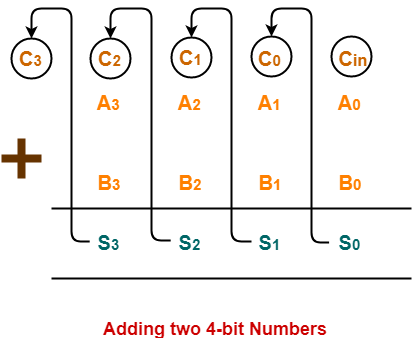Using ripple carry adder, this addition is carried out as shown by the following logic diagram-As shown-

• Ripple Carry Adder works in different stages.
• Each full adder takes the carry-in as input and produces carry-out and sum bit as output.
• The carry-out produced by a full adder serves as carry-in for its adjacent most significant full adder.
• When carry-in becomes available to the full adder, it activates the full adder.
• After full adder becomes activated, it comes into operation.

## Working Of 4-bit Ripple Carry Adder-

Let-

• The two 4-bit numbers are 0101 (A3A2A1A0) and 1010 (B3B2B1B0).
• These numbers are to be added using a 4-bit ripple carry adder.

4-bit Ripple Carry Adder carries out the addition as explained in the following stages-

## Stage-01:

• When Cin is fed as input to the full Adder A, it activates the full adder A.
• Then at full adder A, A0 = 1, B0 = 0, Cin = 0.

Full adder A computes the sum bit and carry bit as-

### Calculation of S0–

S0 = A0 ⊕  B0 ⊕ Cin

S0 = 1 ⊕ 0 ⊕ 0

S0 = 1

### Calculation of C0–

C0 = A0B0 ⊕  B0Cin ⊕ CinA0

C0 = 1.0 ⊕ 0.0 ⊕ 0.1

C0 = 0 ⊕ 0 ⊕ 0

C0 = 0

## Stage-02:

• When C0 is fed as input to the full adder B, it activates the full adder B.
• Then at full adder B, A1 = 0, B1 = 1, C0 = 0.

Full adder B computes the sum bit and carry bit as-

### Calculation of S1–

S1 = A1 ⊕  B1 ⊕ C0

S1 = 0 ⊕ 1 ⊕ 0

S1 = 1

### Calculation of C1–

C1 = A1B1 ⊕  B1C0 ⊕ C0A1

C1 = 0.1 ⊕ 1.0 ⊕ 0.0

C1 = 0 ⊕ 0 ⊕ 0

C1 = 0

## Stage-03:

• When C1 is fed as input to the full adder C, it activates the full adder C.
• Then at full adder C, A2 = 1, B2 = 0, C1 = 0.

Full adder C computes the sum bit and carry bit as-

### Calculation of S2–

S2 = A2 ⊕  B2 ⊕ C1

S2 = 1 ⊕ 0 ⊕ 0

S2 = 1

### Calculation of C2–

C2 = A2B2 ⊕  B2C1 ⊕ C1A2

C2 = 1.0 ⊕ 0.0 ⊕ 0.1

C2 = 0 ⊕ 0 ⊕ 0

C2 = 0

## Stage-04:

• When C2 is fed as input to the full adder D, it activates the full adder D.
• Then at full adder D, A3 = 0, B3 = 1, C2 = 0.

Full adder D computes the sum bit and carry bit as-

### Calculation of S3–

S3 = A3 ⊕  B3 ⊕ C2

S3 = 0 ⊕ 1 ⊕ 0

S3 = 1

### Calculation of C3–

C3 = A3B3 ⊕  B3C2 ⊕ C2A3

C3 = 0.1 ⊕ 1.0 ⊕ 0.0

C3 = 0 ⊕ 0 ⊕ 0

C3 = 0

Thus finally,

• Output Sum = S3S2S1S0 = 1111
• Output Carry = C= 0

### Why Ripple Carry Adder is Called So?

• The carry out produced by each full adder serves as carry-in for its adjacent most significant full adder.
• Each carry bit ripples or waves into the next stage.
• That’s why, it is called as “Ripple Carry Adder”.

• Ripple Carry Adder does not allow to use all the full adders simultaneously.
• Each full adder has to necessarily wait until the carry bit becomes available from its adjacent full adder.
• This increases the propagation time.
• Due to this reason, ripple carry adder becomes extremely slow.
• This is considered to be the biggest disadvantage of using ripple carry adder.